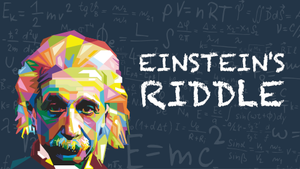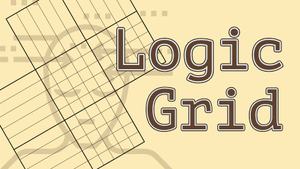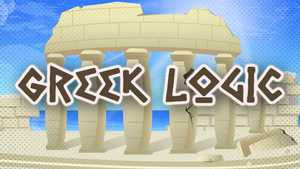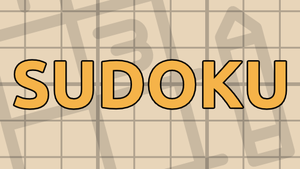1.

• A. 0
• B. 1
• C. 2
• D. 3
• E. 4
2.

The first question whose answer is A is question

• A. 1
• B. 2
• C. 3
• D. 4
• E. there are no "A"s
3.

• A. C
• B. D
• C. E
• D. A
• E. B
4.

• A. C
• B. B
• C. A
• D. E
• E. D

#### How to Play

• Click once on an answer to mark it as wrong;
• Click twice to mark it as correct;
• The bar next to each question shows if the question is logically correct (green) or wrong (red).

• Each test has some number of multiple choice questions about itself;
• There is only one correct answer for each question;
• The solution can be found "without too much trial-and-error".

### Need help?

Is it too hard or you don't understand how to play? Try to solve this one:

### More games##### Einstein's Riddle

Einstein said that only 2% of the world could solve this problem. Can you do it?##### Logic Grid Puzzles

Complete the grid by using logic and the given clues of each problem.##### Greek Logic

Fill the grid using the Greek letters.##### Sudoku

Play the classic japanese logic game.